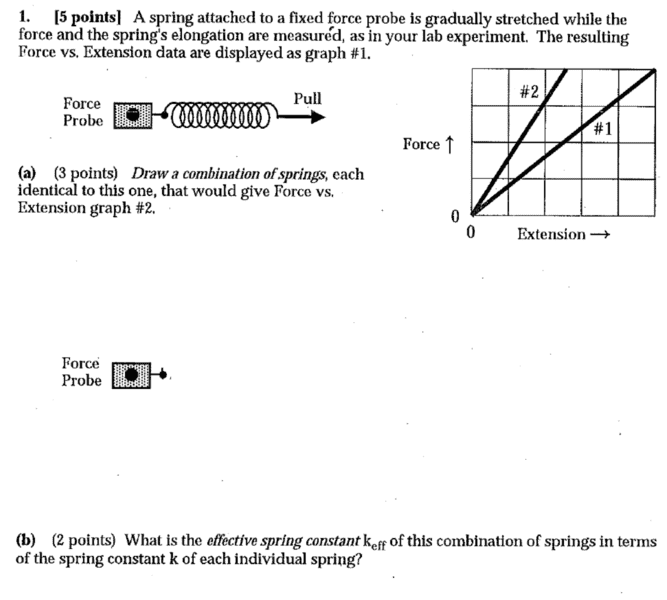# Combining springs to match Force vs Extension Graph

• srekai

## Homework Statementf

## Homework Equations

Hooke's Law: F = -kx
Series spring combinations: ##\frac{1}{k_{eq}} = \frac{1}{k_1}+\frac{1}{k_2}##
Parallel spring combinations: ##k_{eq} = k_1+k_2##

## The Attempt at a Solution

The slope of 1 is ##\frac{4}{5}## and the slope of 2 is ##\frac{3}{2}##

I calculated that we would need ##\frac{15}{8}## times of the original spring to produce the same amount of force. Unfortunately that's just less than 2, so I can't just make it a parallel set of 2 springs.
So I know that in order to get a k of ##\frac{3}{2}## it must be a combination of both parallel and series springs.

So I set it up as such ## \frac{3}{2} = \frac{1}{\frac{4}{5}} + \frac{1}{\text{some parallel combination of springs}}##

I get it so that it would be 5 parallel springs in series with a lone spring.
Does that logic sound correct? And the k_eff of the combination would just be ##\frac{3}{2}##?

#### Attachments

srekai said:
the slope of 2 is ##\frac{3}{2}##
Looks like a little more to me.

If you draw horizontal lines on the graph representing constant force, the extension for #2 looks to be about half #1.